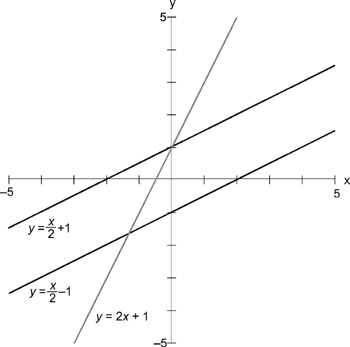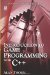3.12 Equations of Lines

3.12 Equations of Lines

There is a direct relationship between algebra and geometry in that it's possible to represent spaces, lines, and shapes using equations. Often, the x and y axes of a coordinate space form the variables of an equation where their values are dependent upon constants and other literal expressions.

Both sides of the equation must be equal, and y is said to be equal to x. Thus, when plotting a point on the graph according to this equation, if x = 1, then y must also be 1, giving the point (1,1). Likewise, if x = 5, then y must also equal 5, giving the point (5,5). If all the possible points that satisfy this equation could be plotted on a graph, they would form the line shown. In other words, every point on this line satisfies the equation y = x. Thus, this equation represents a line, hence, the name linear equation.

 Note You can determine whether a point rests on a line by using the line's equation. Substitute the x and y coordinates for the equation variables. If the two sides of the equation balance, then the point is on the line. Otherwise, the point is not on the line.

3.12.1 X and Y Intercept

It is implied that y = x is not the only equation that can draw lines. All other linear equations can form lines too, such as y = x 5. This will also draw a line. The point where a line intercepts the x axis (where y = 0) is called the x intercept, and the point where a line intercepts the y axis (where x = 0) is called the y intercept.

Thus, given the equation y = x 5, calculate the x intercept. In order to find the point that intercepts the x axis, y must be 0. So, substitute 0 for y.

• x 5 = 0

So:

• x = 5

Therefore (5,0) is the x intercept.

Given the same equation, where is the y intercept? The y intercept is the coordinate where x is 0. So:

• y = x 5

The y intercept will be 5.

In short, wherever an equation of a line is written as y = x + b, b will always be the y intercept.

From most lines plotted on a graph, a right triangle can be formed. The diagonal of this triangle represents the most direct route from point A to point B. If the diagonal is avoided, then in order to travel from A to B we must travel some distance along the x axis and then up or down on the y axis. This route represents the other two sides of the right triangle.

 Note On a graph, you always move along x first and then y.

Consider the two lines in Figure 3.25. Each line has a slope, or gradient. Lines angling to the left are said to have negative slope. Lines inclining to the right have positive slopes. To calculate the slope of a line, the length of the opposite side of the right triangle is divided by the adjacent side. Thus, given two coordinates for any two points on the line, the slope can be determined.Figure 3.25

The gradient of a line is the same as the tangent ratio (tan = opposite / adjacent) for a right triangle. For this reason, a line with a slope of 1 has an angle of elevation of 45 degrees since tan1=45.

3.12.3 Slope Intercept Equation

The equation for a straight line is y = mx + b, where m is the slope of a line and b is the y intercept of a line. Thus, if the slope is 2 and the y intercept is 5, then the equation will be y = 2x + 5.

 Note Two lines that are parallel have the same slope. Two lines are perpendicular if the product of their slopes equals −1.Introduction to Game Programming with C++ (Wordware Game Developers Library)
ISBN: 1598220322
EAN: 2147483647
Year: 2007
Pages: 225
Authors: Alan Thorn

Similar book on Amazon